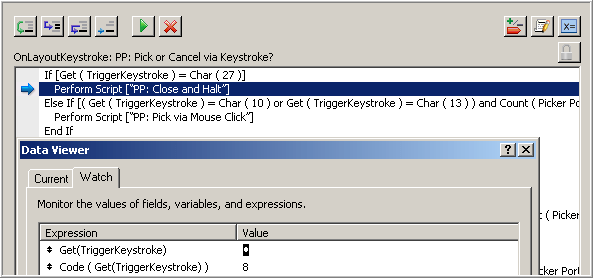# Space Is The Place: a conversation with Jason DeLooze

Recently I asked Jason DeLooze about something that was puzzling me. As usual I found his comments illuminating, and with his permission, am sharing them here.

KF: Why does the following evaluate as true (i.e., return a 1)?

`GetAsNumber("") < 0`

JDL: There actually is method in this madness. FileMaker numbers, dates, times, and timestamps have a duality nature. Remember that FMP stores these data types as text strings and interprets their value in the proper “space” when needed; that is, in their “text” form when the comparison operator is evaluated in “text space”; as a number value when the comparison operator is evaluated in “number space”, etc.

Also bear in  mind that comparison operators act differently in different “spaces”.  For example, the “=” operator has one form in “text space”, another form in “number space”, etc.

KF: Why “etc.”?

JDL: There are “time”, “date” and “timestamp” spaces as well, but let’s not get into those now.

KF: So the reason `GetAsNumber("") < 0` evaluates as true…

JDL: …is because` GetAsNumber("")` is the empty text constant `""`.

For most values and data types, GetAsNumber will return a number-type value. For example, for text type values such as “x” or “x15A3”, GetAsNumber will return the numeric values 0 (zero) and 153, respectively. However, for certain text-type values, such as an empty text value `""` or the text value “?”, GetAsNumber does not return a numeric value; it returns the `""` and ?, respectively.

Once we realize that `GetAsNumber("")` is a text-type value, we also realize that the “<” operator is a “text operator” and the comparison is performed in “text space”. In other words,

`GetAsNumber("") < 0`

is really

`"" < "0"`

which is true.

The true result also happens for the expression

`GetAsNumber(TO::TextField) < 0`

when TO::TextField is empty.

Similar results are observed when using the GetAsDate, GetAsTime, and GetAsTimestamp function on a text field (or value) which is empty.

KF: I wonder if this is related to something I noticed the other day… I had an OnLayoutKeystroke script trigger that was supposed to do something when the user hit Esc, but the Backspace key was also triggering it! This screen shot of the Debugger and the Data Viewer clearly shows FileMaker equating Esc (code 27) with Backspace (code 8).Incidentally, I was able to fix the problem by changing the initial “If” test to

`Code(Get(TriggerKeystroke)) = 27`

…but why did the original “If” test evaluate as true?

JDL: For the same reason that

`Char(8) = Char(27)`

returns true. With the expression

`Get(TriggerKeystroke) = Char(27)`

you are asking FMP to perform the comparison as “text” strings … in “text space”. In text space, certain non-printable characters (such as Esc and Backspace) are lumped together. The same holds true for normal text characters, such as the letter “a” [Char(97)]. For example, the expression

`Get(TriggerKeystroke) = Char(97)`

will evaluate True for both “a” and “A”. In other words, the expression``` ```

`Char(97) = Char(65)`

` `

is True in text space. But if we want to prove these characters are not truly identical, we can use Exact, like so

`Exact ( Char(97) ; Char(65) )`

or more simply``` ```

`Exact ( "a" ; "A" )`

` `

The point is, if you really want to trap for only a certain character, e.g., Esc or “a”, you need to perform the comparison in “number space”. That is why the expression

`Code(Get(TriggerKeystroke)) = 27`

evaluates to true only if the Esc key is pressed. So, when trapping keystrokes, the form of the expression to use

`Get(TriggerKeystroke) = Char(##)`

or

`Code(Get(TriggerKeystroke)) = ##`

depends on your intent. If you want “A” and “a” to be equivalent (or certain non-printable characters to be equivalent), then use the 1st form; if you want to trap for an individual key only, use the 2nd form.

KF: That makes perfect sense. I really appreciate you taking the time to explain that. Any other comments?

JDL: As a general rule of thumb when coding IF and CASE [logical] expressions, always ask yourself “in which comparison world will the expression be evaluated — text, number, date, time, time stamp?” — keeping in mind what happens when the comparison operands are of different types.

KF: Jason, thanks so much.

JDL: My pleasure.

## 3 thoughts on “Space Is The Place: a conversation with Jason DeLooze”

1. At first this sounds reasonable. But look, put this in the Data Viewer:
``` Let( [ x = GetAsNumber ( "x" ) ; // any alpha char. y = GetAsNumber ( 0 ) ; // zero z= GetAsNumber ( "" ) // blank ]; x = y )```

Now change the result to x=z, then try y=z.
x=y is true
x=z is true
y=z is false

Not cool, FileMaker.

1. Kevin Frank says:

I was thinking of Sun Ra when I chose the title… no doubt it’s a generational thing.

This site uses Akismet to reduce spam. Learn how your comment data is processed.